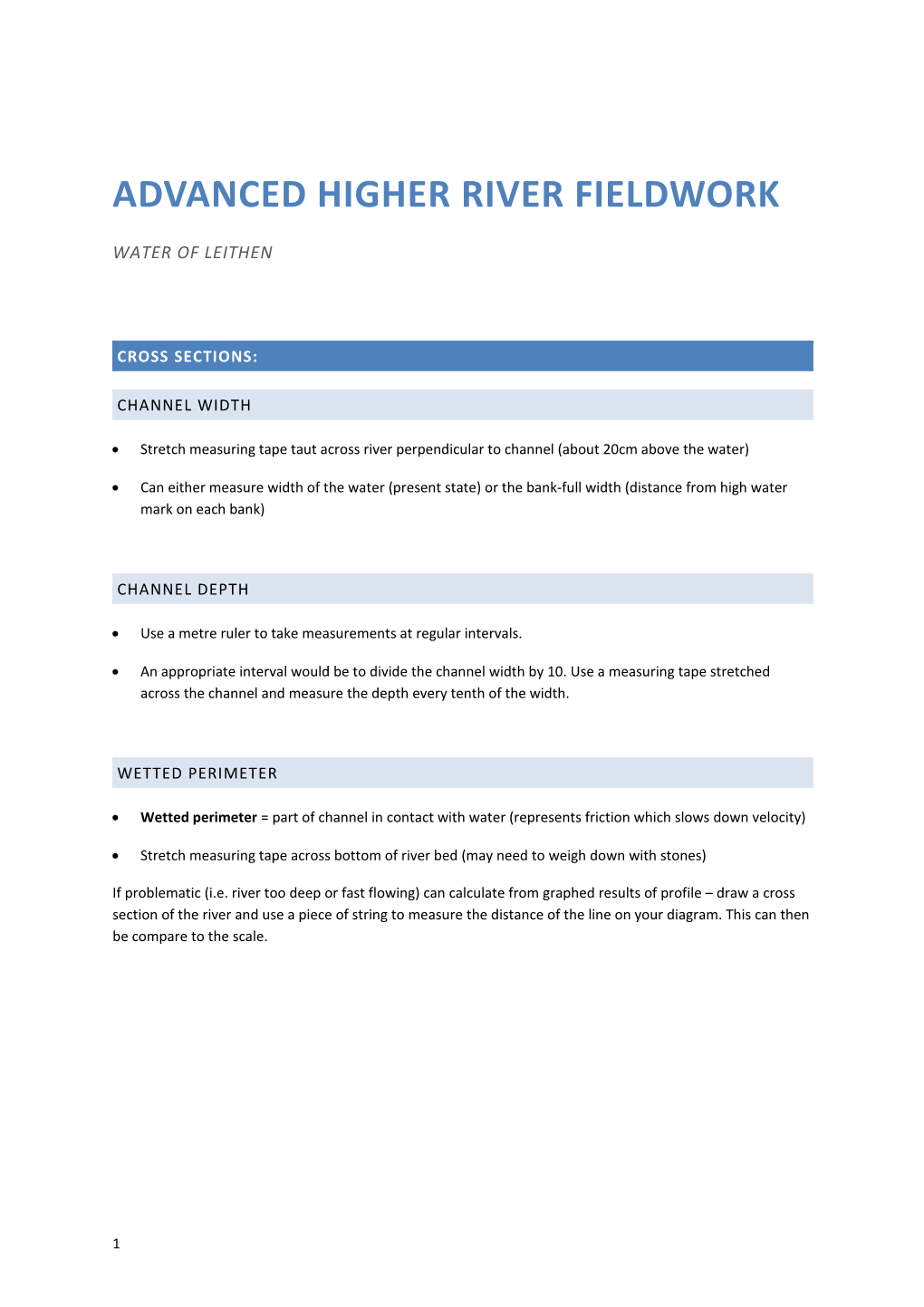Water of Leithen

## Cross sections:

### Channel width

• Stretch measuring tape taut across river perpendicular to channel (about 20cm above the water)
• Can either measure width of the water (present state) or the bank-full width (distance from high water mark on each bank)

### Channel depth

• Use a metre ruler to take measurements at regular intervals.
• An appropriate interval would be to divide the channel width by 10. Use a measuring tape stretched across the channel and measure the depth every tenth of the width.

### Wetted perimeter

• Wetted perimeter = part of channel in contact with water (represents friction which slows down velocity)
• Stretch measuring tape across bottom of river bed (may need to weigh down with stones)

If problematic (i.e. river too deep or fast flowing) can calculate from graphed results of profile – draw a cross section of the river and use a piece of string to measure the distance of the line on your diagram. This can then be compare to the scale.

#### Drawing a cross section

•On graph paper draw the x-axis to scale to represent width, and the y-axis (beneath the x-axis) to represent depth. Make sure the two axes are the same scale.
• From this we can calculate cross-sectional area (width x depth) by counting up the boxes
• We can also calculate the efficiency of the channel – how much of the river is in contact with the river bed/banks, which will result in more friction and reduce velocity

Hydraulic radius = cross-sectional area / wetted perimeter

• This gives indication of river’s ability to maintain energy/transport material. It is an index value (no units) but can be compared to values downstream (higher = more efficient)

## Velocity:

### Float method – surface velocity

• Use an object which floats and is easily visible
• Place 2 ranging poles a set distance apart (10m is probably appropriate)
• Record time taken for object to travel the distance
• Surface velocity = distance / time

To reduce the margin for error be careful when placing the object in the river (don’t throw). Repeat at least 5 times and take an average to improve accuracy.

### Flow meters

• Flow meters will take very accurate readings of velocity
• Take recordings at different widths (e.g. every tenth of the width of the channel) and depths (every 5cm in depth – use the meter stick). This can be done at the same time as measuring channel width/depth.
• Make sure to stand downstream of the flow meter with the propeller pointing upstream – this will ensure you don’t alter the current
• This data can then be used to produce an isovel diagram#### Discharge

Discharge (m3/s) = cross-sectional area (m2) x velocity (m/s)

• Calculates the volume of water that passes in a second

## Sediment analysis:

• Select pebbles (bed load) every tenth of the river width
• To avoid bias select 10 per location. Place hand into river and select first pebble index finger touches (do not look for certain sizes/shapes). Make sure you record from which part of the river each pebble comes (pebble size may vary across the width of a river, especially at meanders)
• Measure the longest axis of each pebble using calipers(or a ruler)
• Compare to sedimentclassification chart or Powers’ visual chart of pebble roundness. Alternatively use a ‘pebbleometer’ if available
• Make sure all pebbles taken out of the river are returned
##### suspended sediment sampler
• Place an open plastic bottle with the top facing upstream and a straw to allow air to leave the bottle
• This will soon fill up with river water travelling downstream
• Leave to stand until any sediment the river is carrying (suspended load) has settled. This can then be analysed

## Follow-up – Sinuosity index

• Sinuosity means how much a river meanders

Sinuosity index = channel length (measured along centre of river)/wavelength (straight-line distance between meanders)

• 1.0 = straight river / above 1.5 = meandering / above 3.0 = tortuous
•This can be calculated using OS maps/Digimaps
###### Project ideas
• Comparisons can be drawn between data collected from different sections of same river (i.e. compare to model) or different rivers
• Gradient can be examined (long profile) – can relate to info on velocity, bedload/sediment etc.
• One river can be investigated or two or more compared
• Human influence on discharge can be investigated by examining catchment characteristics
• Changes in sediment/bed-load along river length
• Compare sediment changes across a river, particularly meanders
• To compare rivers (different locations/environments/rock type in catchment/human interference)
###### Resources:
• Ranging poles
• Tape measures
• Oranges
• Flow meters
• Metre ruler
• Stop watch
• Plastic bottle + straw
• GPS

1